## Introduction

The application of network science to problems in quantum physics is a relatively new and rapidly developing field1. Quantum networks, where the links between nodes represent entangled qubits2,3,4,5,6, are expected to form the basis of the quantum internet. Recent advances in quantum repeater technology have made long-distance, noise-resilient quantum communication possible7,8,9,10,11. These networks have quantum correlations that can be exploited by performing specific local measurements on any node.

Protocols for quantum communication rely on the conservation of correlations in entangled states, and the generation and distribution of entanglement are necessary for quantum networks12. For a given network topology, we want to determine the minimum amount of entanglement necessary between qubits to maintain a giant component in the network, which is a problem analogous to percolation on classical networks13,14,15,16. However, there are crucial differences between classical and quantum networks, limiting the extent to which we can map a classical percolation theory to quantum networks. For example, in a classical random network with N nodes, if an edge between nodes exists with probability p, a subgraph with n nodes and l edges exists above a critical threshold of p given by pcNn/l17. For a quantum network, however, pc ~ N−2 for all subgraphs for large N18. We can also use measurement strategies to alter the topology of a quantum network, meaning that the optimal entanglement percolation threshold needs to be minimized over all possible measurement strategies3.

Quantum networks6 are used to model a scalable communication network based on quantum teleportation. It consists of nodes that denote a local set of qubits and edges, which represent a bipartite and entangled state of qubits shared between the two connected nodes (Fig. 1). The simplest practical quantum network can be built from quantum repeaters19,20 which share only one entangled pair between nodes5. Quantum communication networks are expected to have several advantages over classical communication networks, including the ability to use quantum cryptography and send “quantum software”2,6.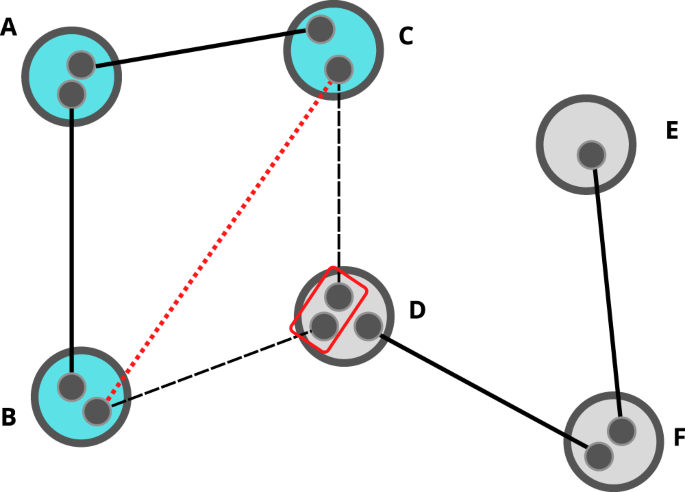Fig. 1: Schematic representation of a quantum network.

The bipartite state of any entangled qubits in the network can be defined as

$$\left|{{\Psi }}\right\rangle \,=\,\cos \theta \left|00\right\rangle +\sin \theta \left|11\right\rangle$$
(1)

up to a unitary transformation, where θ can change from 0 to π/4. Each entangled pair can be converted to a maximally entangled pair with a certain probability p, known as the singlet-conversion probability (SCP), given by $$p\,=\,2{\sin }^{2}\theta$$21. This represents the probability of establishing a perfect communications channel18. Converting every entangled pair in the network to a singlet is equivalent to a bond-percolation process and this measurement strategy is called classical entanglement percolation (CEP)3,13.

Having established the mapping to percolation, it is natural to ask what is the minimum level of entanglement necessary for the formation of a perfect communication channel between any two stations. Using classical-percolation arguments, we can establish the minimum level of entanglement necessary for establishing an infinite cluster under CEP for some simple cases. For example, it is θth = π/4 for 1D chains and θth = π/6 for 2D square lattices3.

Unfortunately, CEP does not give us the lowest possible percolation threshold value for a quantum network because it is possible to lower the entanglement threshold necessary for creating an infinite cluster by changing the network’s topology. This is done through a process known as entanglement swapping, shown in Fig. 1, where two previously unentangled qubits are entangled using local operations and classical communication (LOCC)22.

The network topology may be altered to lower the percolation threshold before converting every link to a singlet by performing a series of entanglement swapping operations. This strategy is known as quantum entanglement percolation (QEP)3,23. The limitation of QEP is that it is not generally adaptable to arbitrary network topology as CEP is. For most network topologies, QEP cannot improve the percolation threshold in general. Note also that neither CEP nor QEP are optimal, meaning that it is impossible to determine if any given measurement strategy results in the lowest potential value of the percolation threshold1. A new local statistical theory, concurrence percolation theory (ConPT), has recently been proposed to explain the observed quantum advantage in quantum networks over the prediction of classical percolation theory24. This theory is analogous to classical percolation theory but fundamentally different, as it is not built on probability measure p but a new variable c for each link, which stands for concurrence—a key measure of bipartite entanglement25. However, ConPT can be computationally very expensive24.

Here, we present a fast and tangible solution for calculating the ConPT threshold. Our method relies on two approximations. The first is the parallel approximation, which treats all paths in the network as non-overlapping. The second is what we call the Sm approximation, where we calculate the total concurrence between nodes using a subset of paths consisting of the m-th shortest paths on the network, with m = 1 referring to the shortest paths. We find that our approximate method agrees closely with the analytical results provided by Meng et al.24. Depending on the choice of Sm, the computation based on this method can be several orders of magnitude faster than the analytical approach. By combining our method with combinatorial expressions for shortest and second-shortest paths we can calculate, for the first time, an approximation for the concurrence for much larger networks than would be analytically possible. Here, we calculate the sponge-crossing concurrence for 2D lattices with up to 2002 nodes. We also extend the notion of concurrence to networks without boundaries and present results for Erdős–Rényi and Barabási–Albert networks of up to 104 nodes. Our results are summarized in Table 1.

## Results and discussion

### Concurrence percolation theory

For pure bipartite states, the concurrence is defined as26

$$c\,=\,\sqrt{2(1 -{{{{{{{\rm{Tr}}}}}}}}{\rho }_{r}^{2})},$$

where ρr is the reduced density matrix of one party (subsystem) of the bipartite state. For qubits, Eq. (1), the concurrence is simply

$$c\,=\,2\cos \theta \sin \theta .$$

Meng et al. then use this quantity in place of probability to construct a concurrence percolation theory (ConPT) on arbitrary network topologies24.

To be more specific, recall that for classical bond percolation on a lattice, any link in the lattice is active with probability p—a variable that should be considered as the link weight. We may then define a “sponge-crossing” quantity, PSC, as the probability that at least one path connecting the two distant boundaries is fully active. As a function of p, PSC can be calculated by summing up all paths that connect the two boundaries of the lattice, following basic addition and multiplication rules of probability measures13. Essentially, we treat PSC as a “weighted sum of all paths”.

Now, given an n-node quantum network, $${{\mathbb{G}}}_{\theta }(n)$$, where all the link weights are θ, by the CEP/QEP schemes we have the mapping $$p\,\equiv\, 2{\sin }^{2}\theta$$ (i.e., the SCP). From classical percolation theory, it is known that a minimum value of p exists, below which the sponge-crossing probability, PSC, becomes zero in the thermodynamic limit n → :

$${p}_{{{{{{{{\rm{th}}}}}}}}}\,=\,\inf \left\{p\,\subset\, [0,1]| {\lim }_{n\,\to\, \infty }{P}_{{{{{{{{\rm{SC}}}}}}}}}[{{\mathbb{G}}}_{\theta }(n)] \, > \, 0\right\}.$$
(2)

This minimum value, pth, is known as the percolation threshold.

The ConPT is constructed differently, using the mapping $$c\,\equiv\, \sin 2\theta$$ instead24. Still, an analogous quantity, CSC, referred to as the sponge-crossing concurrence can be defined as the weighted sum of all paths in terms of this new weight c24. It is believed that a nontrivial threshold on c also exists:

$${c}_{{{{{{{{\rm{th}}}}}}}}}\,=\,\inf \left\{c\,\subset\, [0,1]| {\lim }_{n\,\to\, \infty }{C}_{{{{{{{{\rm{SC}}}}}}}}}[{{\mathbb{G}}}_{\theta }(n)] \, > \, 0\right\},$$
(3)

such that cth is the minimum value of the concurrence c per link, below which CSC becomes zero when n → .

It remains to show how the “weighted sum of paths” is calculated for ConPT. As a problem of path connectivity, the calculation turns out to closely resemble the analysis of an electrical resistor network, where a set of series and parallel rules are needed as the basic connectivity rules24,27. Fundamental quantum communication theorems demand that, for k links connected in series, the total concurrence must be given by

$${c}_{{{{{{{{\rm{seri}}}}}}}}}\,\equiv\, {{{{{{{\rm{seri}}}}}}}}({c}_{1},{c}_{2},\ldots {c}_{k})\,=\,\mathop{\prod }\limits_{i\,=\,1}^{k}{c}_{i}.$$
(4)

The rule for k links connected parallel to each other is more involved, given by

$$\frac{1+\sqrt{1-{c}_{{{{{{{{\rm{para}}}}}}}}}^{2}}}{2}=\max \left\{\mathop{\prod }\limits_{i=1}^{k}\frac{1+\sqrt{1-c_{i}^{2}}}{2},\frac{1}{2}\right\},$$

which yields

$${c}_{{{{{{{{\rm{para}}}}}}}}}\, \equiv\, {{{{{{{\rm{para}}}}}}}}({c}_{1},{c}_{2},\ldots {c}_{k})\,\\ =\,\left\{\begin{array}{ll}2\sqrt{f({c}_{1},\ldots {c}_{k}) -f{({c}_{1}\ldots {c}_{k})}^{2}} &f({c}_{1}\ldots {c}_{k}) \, > \, 1/2,\\ 1\hfill &f({c}_{1}\ldots {c}_{k})\,\le\, 1/2,\end{array}\right.$$
(5)

where $$f({c}_{1}\ldots {c}_{k})\,=\,\mathop{\prod }\nolimits_{i \,=\, 1}^{k}\frac{1 +\sqrt{1 -{c}_{i}^{2}}}{2}$$. A caveat lies in the fact that if the network topology is not series-parallel28 but has nontrivial loops (e.g., a bridge-circuit topology), then CSC cannot be calculated using only series and parallel rules. Higher-order connectivity rules are needed, of which general forms are unknown. There is, however, a heuristic way to approximate these higher-order rules: by employing the so-called star-mesh transform, all possible higher-order rules can be approximated using only the series and parallel rules24.

Equations (4), (5), together with the star-mesh transform, allow us to calculate the “weighted sum of paths” between arbitrary two nodes in a quantum network of arbitrary topology. Formally, we denote the two nodes as the source node (s) and the target node (t), respectively, and we define the final concurrence between them as the s-t concurrence, Cst. Note that although s and t are named differently, they are symmetric and exchangeable. Hence, between any two nodes, a Cst can be calculated by the connectivity rules mentioned above (see Fig. 2 for example).Fig. 2: Demonstration of calculating the s-t concurrence Cst.

On regular lattices, the sponge-crossing concurrence CSC can be calculated by contracting two separate boundaries into two “mega” nodes24 and calculate the s-t concurrence between them. As we increase the network size n, a threshold cth will emerge, accompanied with a sudden jump of CSC as soon as the concurrence c per link becomes larger than cth. This observation supports the existence of ConPT. Also, the observed cth is significantly smaller than all previously known classical-percolation-theory-based schemes24, exhibiting in large-scale quantum networks a quantum advantage that is purely structural.

Despite the fresh insights the ConPT has offered, it has two main limitations:

1. 1.

The heuristic approximation (star-mesh transform) used for higher-order connectivity rules is a double-recursive process that is computationally intensive, thus only feasible for networks of very small size.

2. 2.

Although an s-t concurrence can be calculated between any two nodes in any network topology, the sponge-crossing concurrence CSC is only defined for regular lattices that have apparent boundaries, and thus so is cth. It is unknown how to define cth on complex network topology where we cannot define a boundary, and, provided a proper definition, how (non)trivial the numerical result of cth would be.

Unlike cluster-based percolation theories, ConPT is based on path connectivity, which is arguably more general24. This is why cth simply cannot be defined by clusters like in classical percolation theory for complex network topology. A proper definition and feasible calculation of cth will be of great interest for the theory itself as well as for its applications. Below, we will show our suggested solution that can satisfactorily handle these two limitations of ConPT.

### A fast and tangible solution for concurrence percolation

We start by generalizing CSC from being defined between two apparent boundaries to two arbitrary sets of nodes, denoted S and T. It is reasonable that Eq. (3) will yield a nontrivial ConPT threshold cth for this generalized CSC, as long as the lengths of all paths connecting S and T increase with the network size n. We contract the two sets S and T into two “mega” nodes, which amounts to erasing the internal network topologies of S and T, and then calculate the s-t concurrence between them. This provides us a definitive way of calculating CSC for arbitrary network topology and inferring cth from Eq. (3).

Our numerical computation of cth (“Fast ConPT computation”) on large-scale quantum networks is further made possible by introducing two key simplifying approximations: the parallel approximation and the Sm approximation

#### Parallel approximation

In this Section, we introduce the parallel approximation, where we treat all paths connecting nodes of interest to be parallel, i.e., we treat them as if they have no shared edges. For an arbitrary network with n nodes and uniform edge-weights c, the parallel approximation $${C}_{{{{{{{{\rm{SC}}}}}}}}}^{\prime}$$ of the true sponge-crossing concurrence between two sets of nodes, S and T, is given by

$$\frac{1 +\sqrt{1 -{C}_{{{{{{{{\rm{SC}}}}}}}}}^{{}^{\prime}2}}}{2}\,=\,\max \left\{\mathop{\prod }\limits_{l\,=\,1}^{n}{\left(\frac{1 +\sqrt{1 -{c}^{2l}}}{2}\right)}^{{N}_{l}},\frac{1}{2}\right\},$$
(6)

where Nl is the total number of self-avoiding paths of length l that connect s and t for all sS and tT, respectively. Equation (6) is the mathematical statement of the parallel approximation, indicating that we are taking each of the Nl paths to be parallel (Fig. 3). We illustrate the approximation with a simple example, and then show that on series-parallel networks28 the concurrence calculated under the parallel approximation forms an upper bound to the true concurrence. First, we consider the case where our network is essentially parallel, i.e., it can be expressed as the parallel combination of k subnetworks each with concurrence ci. In this case, the parallel approximation is exact:

$${C}_{{{{{{{{\rm{SC}}}}}}}}}^{\prime}\,=\,{C}_{{{{{{{{\rm{SC}}}}}}}}}\,=\,{{{{{{{\rm{para}}}}}}}}({c}_{1},{c}_{2},\ldots {c}_{k}).$$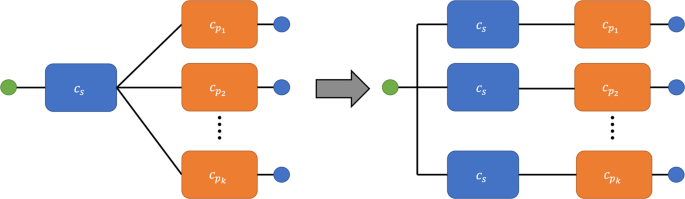Fig. 3: A schematic representation of the parallel approximation.

The more interesting case is that of an essentially series network, i.e., a network that can be decomposed as a combination of subnetworks in series. We consider an exemplary network that splits into k branches, each with concurrence $${c}_{{p}_{i}}$$ (Fig. 3). The concurrence of the segment before branching is cs. Following the series and parallel rules (Eqs. 4, 5), the sponge-crossing concurrence from the left of this network segment to the right is

$${C}_{{{{{{{{\rm{SC}}}}}}}}}=\left\{\begin{array}{ll}{c}_{s}\left(2\sqrt{f({c}_{{p}_{0}},\ldots {c}_{{p}_{k}}) -f{({c}_{{p}_{0}},\ldots {c}_{{p}_{k}})}^{2}}\right) &f({c}_{{p}_{0}},\ldots {c}_{{p}_{k}}) \, > \, 1/2,\\ {c}_{s}\hfill &f({c}_{{p}_{0}},\ldots {c}_{{p}_{k}})\,\le\, 1/2,\end{array}\right.$$

where $$f({c}_{{p}_{0}},\ldots {c}_{{p}_{k}})\,=\,\mathop{\prod }\nolimits_{i \,=\, 1}^{k}g({c}_{{p}_{i}})\,=\,\mathop{\prod }\nolimits_{i \,=\, 1}^{k}\left(\frac{1 +\sqrt{1 -{c}_{{p}_{i}}^{2}}}{2}\right)$$. Under the parallel approximation, the network is transformed so that the concurrence of the segment is given by

$${C}_{{{{{{{{\rm{SC}}}}}}}}}^{\prime}\,=\,\left\{\begin{array}{ll}2\sqrt{f({c}_{s}{c}_{{p}_{0}},\ldots {c}_{s}{c}_{{p}_{k}}) -f{({c}_{s}{c}_{{p}_{0}},\ldots {c}_{s}{c}_{{p}_{k}})}^{2}}&f({c}_{s}{c}_{{p}_{0}},\ldots {c}_{s}{c}_{{p}_{k}}) \, > \, 1/2,\\ 1\hfill &f({c}_{s}{c}_{{p}_{0}},\ldots {c}_{s}{c}_{{p}_{k}})\,\le\, 1/2.\end{array}\right.$$

Since $${c}_{s}{c}_{{p}_{i}}\,\le\, {c}_{{p}_{i}}$$, it follows that $$g({c}_{s}{c}_{{p}_{i}})\,\ge\, g({c}_{{p}_{i}})$$ and $$f({c}_{s}{c}_{{p}_{0}},\ldots {c}_{s}{c}_{{p}_{k}})\,\ge\, f({c}_{{p}_{0}},\ldots {c}_{{p}_{k}})$$. There are three cases:

1. 1.

$$1/2\,\ge\, f({c}_{s}{c}_{{p}_{0}},\ldots {c}_{s}{c}_{{p}_{k}})\,\ge\, f({c}_{{p}_{0}},\ldots {c}_{{p}_{k}})$$. In this case, it is obvious that $${C}_{{{{{{{{\rm{SC}}}}}}}}}^{\prime}\,=\,1\,\ge\, {C}_{{{{{{{{\rm{SC}}}}}}}}}$$.

2. 2.

$$f({c}_{s}{c}_{{p}_{0}},\ldots {c}_{s}{c}_{{p}_{k}})\,\ge\, f({c}_{{p}_{0}},\ldots {c}_{{p}_{k}}) \, > \, 1/2$$. Now we consider

$${\left(\frac{{C}_{{{{{{{{\rm{SC}}}}}}}}}^{\prime}}{{C}_{{{{{{{{\rm{SC}}}}}}}}}}\right)}^{2}\,=\,\frac{f({c}_{s}{c}_{{p}_{0}},\ldots {c}_{s}{c}_{{p}_{k}})(1 -f({c}_{s}{c}_{{p}_{0}},\ldots {c}_{s}{c}_{{p}_{k}}))}{{c}_{s}^{2}f({c}_{{p}_{0}},\ldots {c}_{{p}_{k}})(1 -f({c}_{{p}_{0}},\ldots {c}_{{p}_{k}}))}.$$

We would like to show that the above expression is no less than unity by considering some limiting cases. When $${c}_{{p}_{i}}\,=\,0$$ for all i it results in $${C}_{{{{{{{{\rm{SC}}}}}}}}}\,=\,{C}_{{{{{{{{\rm{SC}}}}}}}}}^{\prime}\,=\,0$$. If we increase the concurrence of a single branch i to be greater than zero while holding the other branches to be zero, then the expression becomes

$$\frac{\left(\frac{1}{2} +\frac{1}{2}\sqrt{1 -{c}_{s}^{2}{c}_{{p}_{i}}^{2}}\right)\left[1 -\left(\frac{1}{2} +\frac{1}{2}\sqrt{1 -{c}_{s}^{2}{c}_{{p}_{i}}^{2}}\right)\right]}{{c}_{s}^{2}\left(\frac{1}{2} +\frac{1}{2}\sqrt{1 -{c}_{{p}_{i}}^{2}}\right)\left[1 -\left(\frac{1}{2} +\frac{1}{2}\sqrt{1 -{c}_{{p}_{i}}^{2}}\right)\right]}\,=\,1,$$

or $$g({c}_{s}{c}_{{p}_{i}})(1 -g({c}_{s}{c}_{{p}_{i}}))\,=\,{c}_{s}^{2}g({c}_{{p}_{i}})(1 -g({c}_{{p}_{i}}))$$. This is simply to state that, given only one branch, the parallel approximation is exact (since there is one and only one path). We now add a second branch, j, while leaving the remaining k − 2 branches with zero concurrence. Now we have

$${\left(\frac{{C}_{{{{{{{{\rm{SC}}}}}}}}}^{\prime}}{{C}_{{{{{{{{\rm{SC}}}}}}}}}}\right)}^{2}\,=\,\frac{g\big({c}_{s}{c}_{{p}_{i}}\big)g\big({c}_{s}{c}_{{p}_{j}}\big)\left(1 -g\big({c}_{s}{c}_{{p}_{i}}\big)g\big({c}_{s}{c}_{{p}_{j}}\big)\right)}{{c}_{s}^{2}g\big({c}_{{p}_{i}}\big)g\big({c}_{{p}_{j}}\big)\left(1 -g\big({c}_{{p}_{i}}\big)g\big({c}_{{p}_{j}}\big)\right)}.$$

For cs → 0, the expression above reduces to

$$\mathop{\lim }\limits_{{c}_{s}\to 0}{\left(\frac{{C}_{{{{{{{{\rm{SC}}}}}}}}}^{\prime}}{{C}_{{{{{{{{\rm{SC}}}}}}}}}}\right)}^{2} =\; \frac{2{c}_{{p}_{i}}^{2} +2{c}_{{p}_{j}}^{2}}{{c}_{{p}_{i}}^{2} +{c}_{{p}_{j}}^{2} -\frac{1}{2}{c}_{{p}_{i}}^{2}{c}_{{p}_{j}}^{2} +{c}_{{p}_{j}}^{2}\sqrt{1 -{c}_{{p}_{i}}^{2}} +{c}_{{p}_{i}}^{2}\sqrt{1 -{c}_{{p}_{j}}^{2}}}\\ \ge \frac{2{c}_{{p}_{i}}^{2} +2{c}_{{p}_{j}}^{2}}{2{c}_{{p}_{i}}^{2} +2{c}_{{p}_{j}}^{2} -\frac{1}{2}{c}_{{p}_{i}}^{2}{c}_{{p}_{j}}^{2}}\,\ge\, 1,$$

and for cs = 1, the expression is equal to unity. Hence, since the derivative of the expression w.r.t. cs,

$$\frac{\partial }{\partial {c}_{s}}{\left(\frac{{C}_{{{{{{{{\rm{SC}}}}}}}}}^{\prime}}{{C}_{{{{{{{{\rm{SC}}}}}}}}}}\right)}^{2}\,=\,-\frac{{c}_{s}{c}_{{p}_{i}}^{2}{c}_{{p}_{j}}^{2}\left(\sqrt{1 -{c}_{s}^{2}{c}_{{p}_{i}}^{2}} +\sqrt{1 -{c}_{s}^{2}{c}_{{p}_{j}}^{2}} +\sqrt{1 -{c}_{s}^{2}{c}_{{p}_{i}}^{2}}\sqrt{1 -{c}_{s}^{2}{c}_{{p}_{j}}^{2}}\right)}{{2}^{3}g({c}_{{p}_{i}})g({c}_{{p}_{j}})\left(1 -g({c}_{{p}_{i}})g({c}_{{p}_{j}})\right)\sqrt{1 -{c}_{s}^{2}{c}_{{p}_{i}}^{2}}\sqrt{1 -{c}_{s}^{2}{c}_{{p}_{j}}^{2}}},$$

is nonpositive between 0 ≤ cs ≤ 1, we derive that $${C}_{{{{{{{{\rm{SC}}}}}}}}}^{\prime}$$ is no less than CSC. This tells us that the addition of another branch results in the approximated concurrence being an upper bound for the true concurrence calculated through series-parallel rules. As more branches are added to the expression, the result can be easily generalized, so that $${C}_{{{{{{{{\rm{SC}}}}}}}}}^{\prime}/{C}_{{{{{{{{\rm{SC}}}}}}}}}\,\ge\, 1$$ remains true.

1. 3.

$$f({c}_{s}{c}_{{p}_{0}},\ldots {c}_{s}{c}_{{p}_{k}}) \, > \, 1/2\,\ge\, f({c}_{{p}_{0}},\ldots {c}_{{p}_{k}})$$. As before, we consider

$${\left(\frac{{C}_{{{{{{{{\rm{SC}}}}}}}}}^{\prime}}{{C}_{{{{{{{{\rm{SC}}}}}}}}}}\right)}^{2}\,=\,\frac{f({c}_{s}{c}_{{p}_{0}},\ldots {c}_{s}{c}_{{p}_{k}})(1 -f({c}_{s}{c}_{{p}_{0}},\ldots {c}_{s}{c}_{{p}_{k}}))}{{c}_{s}^{2}/4}.$$

For cs → 0, the expression reduces to

$$\mathop{\lim }\limits_{{c}_{s}\,\to\, 0}{\left(\frac{{C}_{{{{{{{{\rm{SC}}}}}}}}}^{\prime}}{{C}_{{{{{{{{\rm{SC}}}}}}}}}}\right)}^{2}\,=\,\mathop{\sum }\limits_{i\,=\,1}^{k}{c}_{{p}_{i}}^{2}\,\ge\, 1.$$

In particular, the inequality holds because $$f({c}_{{p}_{1}},\ldots {c}_{{p}_{k}})$$ is a concave function defined on the simplex

$$\left\{\left({c}_{{p}_{1}}^{2},\ldots ,{c}_{{p}_{k}}^{2}\right)| \mathop{\sum }\limits_{i\,=\,1}^{k}{c}_{{p}_{i}}^{2}\,=\,1\right\},$$

where it satisfies $$f({c}_{{p}_{1}},\ldots {c}_{{p}_{k}}) \, > \, 1/2$$ except at the vertices where all but one $${c}_{{p}_{i}}^{2}$$ are equal to zero. Thus, due to the monotonicity of f, one requires $$\mathop{\sum }\nolimits_{i \,=\, 1}^{k}{c}_{{p}_{i}}^{2}\,\ge\, 1$$ to guarantee that $$f({c}_{{p}_{1}},\ldots {c}_{{p}_{k}})\,\le\, 1/2$$. Next, as cs → 1 the function $$f({c}_{s}{c}_{{p}_{1}},\ldots {c}_{s}{c}_{{p}_{k}})$$ will become less than 1/2 and we will revert to case 1. Therefore, there is an upper bound on cs. As cs approaches its upper bound, $$f({c}_{s}{c}_{{p}_{1}}\ldots {c}_{s}{c}_{{p}_{k}})\,\to\, 1/2$$, and thus

$$\mathop{\lim }\limits_{f({c}_{s}{c}_{{p}_{1}}\ldots {c}_{s}{c}_{{p}_{k}})\,\to\, 1/2}{\left(\frac{{C}_{{{{{{{{\rm{SC}}}}}}}}}^{\prime}}{{C}_{{{{{{{{\rm{SC}}}}}}}}}}\right)}^{2}\,=\,\frac{1}{{c}_{s}^{2}}\,\ge\, 1.$$

Since for this case, $$\frac{\partial }{\partial {c}_{s}}{\left(\frac{{C}_{{{{{{{{\rm{SC}}}}}}}}}^{\prime}}{{C}_{{{{{{{{\rm{SC}}}}}}}}}}\right)}^{2}$$ is nonpositive between cs → 0 and the presumed upper bound of cs, again we derive that $${C}_{{{{{{{{\rm{SC}}}}}}}}}^{\prime}/{C}_{{{{{{{{\rm{SC}}}}}}}}}\,\ge\, 1$$.

Finally, since every series-parallel network can be decomposed into essentially series or parallel configurations, taken together we have shown that $${C}_{{{{{{{{\rm{SC}}}}}}}}}^{\prime}$$ is an upper bound for CSC on series-parallel networks. Interestingly, as we will see, this upper bound seemingly becomes tighter as the network becomes larger. We hence expect that a new concurrence threshold on $${C}_{{{{{{{{\rm{SC}}}}}}}}}^{\prime}$$ can emerge, which should numerically approach the true cth from below and match cth in the thermodynamic limit n → .

#### Sm approximation

For most regular lattices and complex networks, however, the distribution of Nl (Eq. 6) is not trivial. When we look at arbitrary networks, the calculation for the sponge-crossing concurrence is essentially a path-counting problem which may require approximation as well.

Although the literature of path counting on graphs is rich and well studied, we are not aware of any closed-form solutions for enumeration of self-avoiding walks of arbitrary length for even the simplest network (like 2D lattices)29. While approximate path enumerations exist for both 2D lattices30 and random networks31, we find them impractical, since the concurrence calculation is very sensitive to Nl for small l. Indeed, observation of Eq. (6) implies that a single path’s contribution to the total concurrence decreases with increasing l and increases with increasing Nl. Even though longer paths (ln) will outnumber shorter paths by several orders of magnitude, shorter paths will still contribute significantly more to the concurrence.

Based on this, if we define Sm as the set which contains up to the m-th shortest paths (i.e., the shortest paths, the 2nd shortest paths, and so on up to the m-th shortest paths) between s and t for all sS and tT, then it is possible to approximate the sponge-crossing concurrence between S and T using only these paths. When $$m\,=\,{m}_{\max }$$, Sm becomes the set of all sponge-crossing paths.

In this Section, using our Fast ConPT computation, we present numerical results for different networks of large size n. We numerically estimate the finite-size ConPT threshold in terms of $${\theta }_{{{{{{{{\rm{th}}}}}}}}}\,\equiv\, \frac{1}{2}{\sin }^{-1}{c}_{{{{{{{{\rm{th}}}}}}}}}$$, determining its position on the critical curve by matching the corresponding sponge-crossing concurrence at the half point, CSC = 1/2.

### Bethe Lattice (Cayley Tree)

Given a finite Bethe lattice (i.e., a Cayley tree) with L layers and coordination number k32,33, all paths from the root node to any one of the boundary nodes have the same length, L. Since only one path exists from the root node to any node on the boundary, the number of paths of length L is

$${N}_{L}\,=\,k{(k -1)}^{L -1}.$$
(7)

There is no need to employ the Sm approximation since all paths are exactly known. Only the parallel approximation $${C}_{{{{{{{{\rm{SC}}}}}}}}}^{\prime}$$ of the sponge-crossing concurrence CSC is to be calculated, which is given by (following Eq. 6)

$$\frac{1 +\sqrt{1 -{C}_{{{{{{{{\rm{SC}}}}}}}}}^{{}^{\prime}2}}}{2}\,=\,\max \left\{{\left(\frac{1 +\sqrt{1 -{c}^{2L}}}{2}\right)}^{{N}_{L}},\frac{1}{2}\right\}.$$
(8)

To solve for cth, near $${C}_{{{{{{{{\rm{SC}}}}}}}}}^{\prime}\,=\,0$$ we let

$${\left(\frac{1 +\sqrt{1 -{c}_{{{{{{{{\rm{th}}}}}}}}}^{2L}}}{2}\right)}^{{N}_{L}}\,=\,1 -\epsilon$$
(9)

given an arbitrarily small positive ϵ. This gives rise to

$${c}_{{{{{{{{\rm{th}}}}}}}}}^{2L}\,=\,1 -{\left[2{\left(1-\epsilon \right)}^{1/{N}_{L}} -1\right]}^{2}\,\simeq\, -4{N}_{L}^{-1}\ln \left(1 -\epsilon \right) +O({N}_{L}^{-2}),$$
(10)

and thus

$${c}_{{{{{{{{\rm{th}}}}}}}}}\,\simeq\, {\left(\frac{4\epsilon }{k}\right)}^{\frac{1}{2L}}{\left(\frac{1}{k -1}\right)}^{\frac{L -1}{2L}}\,\simeq\, \frac{1}{\sqrt{k -1}}$$
(11)

in the limit of large L. This is identical to the exact ConPT threshold calculated by Meng et al.24 using a recursive renormalization trick on the series and parallel rules (Eqs. 4, 5).

Interestingly, it is known that a saturation point csat < 1 also exists in ConPT24, namely, before c reaches unity, CSC will already reach unity at c = csat. This is because of the maximum function appearing in the parallel rule (Eq. 5). It is also obvious that csat ≥ cth, given the monotonicity of the series and parallel rules. To see if we can solve for csat using the parallel approximation too, let

$${\left(\frac{1 +\sqrt{1 -{c}_{{{{{{{{\rm{sat}}}}}}}}}^{2L}}}{2}\right)}^{{N}_{L}}\,=\,\frac{1}{2},$$
(12)

set by $${C}_{{{{{{{{\rm{SC}}}}}}}}}^{\prime}\,=\,1$$. This yields

$${c}_{{{{{{{{\rm{sat}}}}}}}}}^{2L}\,=\,1 -{\left[2{\left(1/2\right)}^{1/{N}_{L}} -1\right]}^{2}\,\simeq\, 4{N}_{L}^{-1}\ln 2 +O({N}_{L}^{-2}),$$
(13)

and thus

$${c}_{{{{{{{{\rm{sat}}}}}}}}}\,\simeq\, {\left(\frac{4\ln 2}{k}\right)}^{\frac{1}{2L}}{\left(\frac{1}{k -1}\right)}^{\frac{L -1}{2L}}\,\simeq\, \frac{1}{\sqrt{k -1}}.$$
(14)

We see that the saturation point calculated using the parallel approximation is equal to cth, which is underestimated, since the true saturation point is given by $${c}_{{{{{{{{\rm{sat}}}}}}}}}\,=\,\sqrt{{(1/2)}^{1/k} -{(1/4)}^{1/k}}/\sqrt{{(1/2)}^{(k -1)/k} -{(1/4)}^{(k -1)/k}}$$24, which can be calculated similarly using a recursive renormalization trick on the series and parallel rules (Eqs. 4, 5).

For validation purposes, numerical results of the sponge-crossing concurrence on the Bethe lattice using the parallel approximation versus the true ConPT results are shown in Fig. 4. We see that as L increases, both cth and csat (where $$c\,=\,2\cos \theta \sin \theta$$) approach $$1/\sqrt{k -1}$$ from below and above, respectively, consistent with our theoretical result. Hence, it is highly suggested that the parallel approximation can correctly estimate the true ConPT threshold cth in the thermodynamic limit.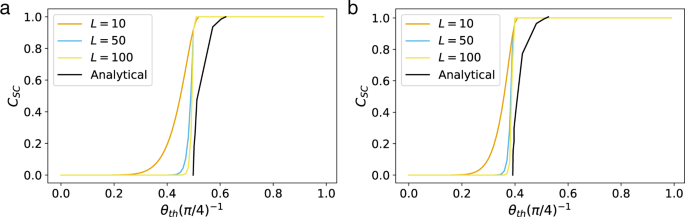Fig. 4: The sponge-crossing concurrence for the Bethe lattice under the parallel approximation.

### 2D square lattices

In a 2D square lattice of n nodes ($$\sqrt{n}\,\in\, {\mathbb{Z}}$$), the length of the mth shortest self-avoiding path, between source and target nodes of coordinates s = (xs, ys) and t = (xt, yt) ($$1\,\le\, {x}_{s},{x}_{t}\,\le\, \sqrt{n}$$ and $$1\,\le\, {y}_{s},{y}_{t}\,\le\, \sqrt{n}$$), is simply

$${l}_{m}\,=\,\left|{x}_{s} -{x}_{t}\right|+\left|{y}_{s} -{y}_{t}\right| +2\left(m -1\right).$$

Now, let S and T denote the left (xs = 1) and right ($${x}_{t}\,=\,\sqrt{n}$$) boundaries. Let s = (1, ys) S and $$t\,=\,(\sqrt{n},{y}_{t})\,\in\, T$$. Under the Sm approximation, the total number of self-avoiding paths of length l between S and T is given by

$${N}_{l}\,\approx\, \mathop{\sum }\limits_{{y}_{s}\,=\,1}^{\sqrt{n}}\mathop{\sum }\limits_{{y}_{t}\,=\,1}^{\sqrt{n}}{\delta }_{{l}_{1}l}{N}_{{l}_{1}}(s\,\to\, t) +{\delta }_{{l}_{2}l}{N}_{{l}_{2}}(s\,\to\, t) +\cdots +{\delta }_{{l}_{m}l}{N}_{{l}_{m}}(s\,\to\, t),$$
(15)

where δij is the Kronecker delta. This approximation of Nl is then substituted into the parallel approximation (Eq. 6) to calculate CSC between S and T.

For m ≤ 2, it is possible to directly enumerate the 1st and 2nd shortest self-avoiding paths between every pair of s and t. The general expressions are given by (w.l.o.g., xs ≤ xt and ys ≤ yt)

$${N}_{{l}_{1}}\left(s\,\to\, t\right)\,=\,\left(\begin{array}{c}\left|{x}_{s} -{x}_{t}\right| +\left|{y}_{s} -{y}_{t}\right|\\ | {x}_{s} -{x}_{t}| \end{array}\right),$$
(16)

and

$${N}_{{l}_{2}}\left(s\,\to\, t\right)= \mathop{\sum }\limits_{{x}^{\prime}\,=\,\max \{{x}_{s},2\}}^{\min \{{x}_{t} +1,\sqrt{n}\}}\mathop{\sum }\limits_{{y}^{\prime}\,=\,{y}_{s}}^{{y}_{t} -2}\left(\begin{array}{c}| {x}_{s} -{x}^{\prime}| +| {y}_{s} -{y}^{\prime}| \\ | {x}_{s} -{x}^{\prime}| \end{array}\right)\left(\begin{array}{c}| {x}_{t} -{x}^{\prime} +1| +| {y}_{t} -{y}^{\prime} -2| \\ | {x}_{t} -{x}^{\prime} +1| \end{array}\right)\\ +\mathop{\sum }\limits_{{x}^{\prime}\,=\,{x}_{s}}^{{x}_{t} -2}\,\,\mathop{\sum }\limits_{{y}^{\prime}\,=\,\max \{{y}_{s},2\}}^{\min \{{y}_{t} +1,\sqrt{n}\}}\left(\begin{array}{c}| {y}_{s} -{y}^{\prime}| +| {x}_{s} -{x}^{\prime}| \\ | {y}_{s} -{y}^{\prime}| \end{array}\right)\left(\begin{array}{c}| {y}_{t} -{y}^{\prime} +1| +| {x}_{t} -{x}^{\prime} -2| \\ | {y}_{t} -{y}^{\prime} +1| \end{array}\right)\\ +{{{{{{{\mathcal{B}}}}}}}}({x}_{s},{x}_{t})\left(\begin{array}{c}| {x}_{t} +1 -{x}_{s}| +| {y}_{t} -1 -{y}_{s}| \\ | {x}_{t} +1 -{x}_{s}| \end{array}\right) +{{{{{{{\mathcal{B}}}}}}}}({y}_{s},{y}_{t})\left(\begin{array}{c}| {y}_{t} +1 -{y}_{s}| +| {x}_{t} -1 -{x}_{s}| \\ | {y}_{t} +1 -{y}_{s}| \end{array}\right),$$
(17)

where the boundary effect

$${{{{{{{\mathcal{B}}}}}}}}(u,v)\,=\,\left\{\begin{array}{ll}0,&u=1,v=\sqrt{n}\hfill\\ 1, &u=1,v \; < \; \sqrt{n}\quad{{{{{{{\rm{or}}}}}}}}\quad u \, > \, 1,v\,=\,\sqrt{n}\\ 2, &u \; > \; 1,v \; < \; \sqrt{n}\hfill\end{array}\right.$$
(18)

is also taken into account. In particular, Eq. (17) is obtained due to the fact that every 2nd shortest self-avoiding path in the square lattice, having length l2 = xs − xt + ys − yt + 2, must contain one and only one of the configurations as shown in Fig. 5. The first and second terms in Eq. (17) account for the two “Z”-shape configurations (Fig. 5a, b), respectively; the third term for the two “L”-shape configurations (Fig. 5c, d); and the last term for the other two “L”-shape configurations (Fig. 5e, f).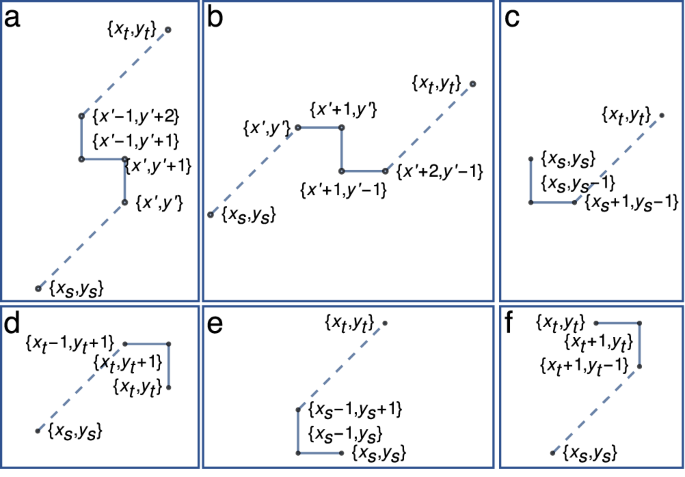Fig. 5: Characterization of second-shortest paths using primitive steps.

#### Piecewise path enumeration algorithm

For m > 2, it becomes difficult to write down a closed-form combinatorial expression like Eqs. (16, 17) for $${N}_{{l}_{m}}(s\,\to\, t)$$. A path enumeration algorithm is thus needed. We treat paths of length lm with m > 2 as deviations from the 1st and 2nd shortest paths. For a given m, these deviations can only take a finite number of shapes. Once we have identified these primitive deviations, we must next identify positions in the lattice where these deviations can be placed. Finally, we count the total number of paths by counting the number of shortest paths between deviations using Eqs. (16, 17).

For example, given source and target nodes s and t, all 3rd-shortest paths (m = 3) have either two single-step deviations or one double-step deviation from the 1st shortest path. For the case where we have two single-step deviations, we first identify two sets of points, D1 and D2, where the first and second deviations can happen respectively. Then we calculate $${N}_{s,{D}_{1}}$$ (the number of shortest paths from s to every point in D1), $${N}_{{D}_{1},{D}_{2}}$$ (the number of shortest paths from every point in D1 to every point in D2), and $${N}_{{D}_{2},t}$$ (the number of shortest paths from every point in D2 to t). The total number of 3rd-shortest paths is then given by $${N}_{{l}_{2}}(s\,\to\, t)\,=\,{N}_{s,{D}_{1}}{N}_{{D}_{1},{D}_{2}}{N}_{{D}_{2},t}$$. This algorithm, while significantly faster than a brute-force path enumeration, is still too involved for large m. We use this algorithm to calculate S3 exclusively.

#### Numerical calculations

The final numerical results of CSC, calculated using the exact combinatorial expressions (S1, S2) and/or the piecewise path enumeration algorithm (S3), are shown in Fig. 6. We can see from Fig. 6a that the transition in the value of the sponge-crossing concurrence becomes sharper as the network size increases. From Fig. 6b–d we see that for large enough m or n, the numerical threshold θth levels out at constant values that are very close to those calculated using the star-mesh transform. For example, for n2 = 8 the Fast ConPT method yields θth = 0.4, compared to the value of θth = 0.416 calculated using the star-mesh transform24. This suggests that our Fast ConPT calculation can yield a good approximation of the ConPT threshold. We can also see from Fig. 7 that the Fast ConPT computation is over 100 times faster than the star-mesh transform method.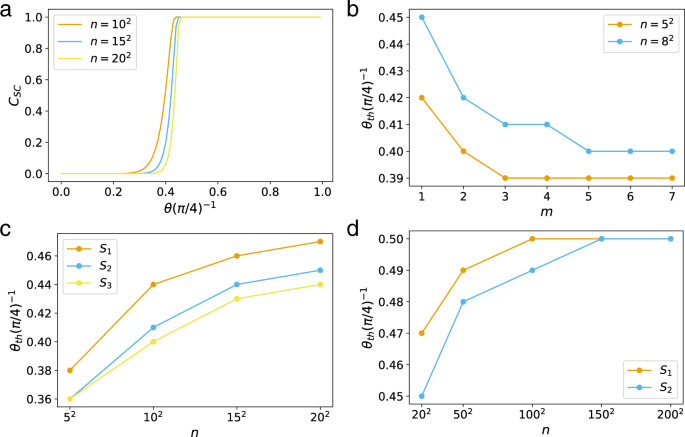Fig. 6: Fast ConPT calculation on 2D square lattices.Fig. 7: The speed-up obtained by the Fast ConPT algorithm over star-mesh reduction.

### Complex network topologies

Unlike 2D square lattices, we cannot write down any analytical expressions for the path-length distribution of complex networks. While techniques to enumerate paths give a good estimate of the total number of paths31, they approximate the path-length distribution poorly. This means that we must enumerate paths through brute-force methods and this restricts our analysis to sparse graphs.

For complex networks, we simply define the sponge-crossing concurrence as the Cst between two nodes s and t which means that S = {s} and T = {t}. We pick s and t such that the shortest path between them is equal to the diameter of the network. In general there might be multiple choices for s and t that meet this criterion, and we randomly choose one of these pairs.

We randomly generate 100 network realizations of a given size and degree distribution and average the concurrence percolation threshold of all networks. These results are reported in Table 1 along with the standard error, $${\sigma }/{\sqrt{N}}$$, where σ is the standard deviation and N = 100 is the number of realizations of each random graph.

#### Erdős–Rényi Network

Results for Erdős–Rényi (ER) networks34 are shown in Figs. 8, 9a, 10a, b. The concurrence is calculated under the S6 approximation for different settings of network sizes and average degrees. The results are averaged over 100 network realizations for each setting. For small values of m, the behavior of the concurrence for ER networks can be approximated with a power-law fit, as shown in Fig. 8. Figure 10a shows that the value of θth converges with increasing network size for smaller values of the average degree, e.g., $$\left\langle k\right\rangle \,=\,4$$. For larger values of the average degree, such as $$\left\langle k\right\rangle \,=\,8$$, we do not see the value of the threshold converging for the same network size.Fig. 8: The concurrence percolation threshold as a function of m for Erdős–Rényi (ER) networks.

The calculation of the number of paths becomes increasingly computationally intensive for larger values of the average degree and we must restrict our analysis to small values of m. Figure 10b shows how the concurrence percolation threshold changes as a function of average degree under the S1 approximation.

#### Barabási–Albert network

Many real-world networks show power-law degree distribution, such as the Internet, WWW, scientific collaboration networks, protein-protein interaction networks, and actor networks14,35. Barabási–Albert (BA) model36 is the first model to describe the structure property of such networks, using preferential attachment. In this model, every new node in the graph is assigned z edges, where z is known as the coordination number, and nodes with higher degrees are more likely to be selected. The classical bond-percolation threshold for a BA network with z > 1 and n →  is pc = 037,38.

Results for BA networks are shown in Figs. 9b, 10c, d. For z = 1 there are no loops in the network and the relatively small number of paths connecting any two nodes allows us to calculate the concurrence for up to 104 nodes, shown in Fig. 10c. We also look at smaller networks with higher coordination numbers, up to z = 25, shown in Fig. 10d. Unlike ER networks, the value of θth decreases with the increasing network size.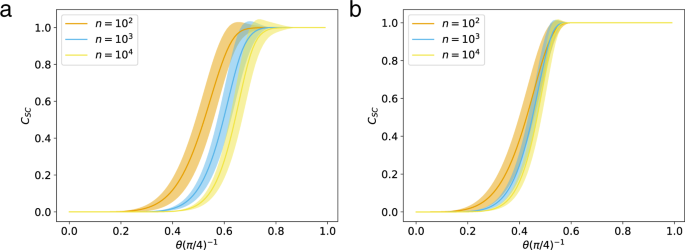Fig. 9: The s-t concurrence as a function of entanglement strength for complex networks.Fig. 10: The Fast ConPT calculation on complex networks.

#### Comparison with Classical Entanglement Percolation (CEP)

As a baseline comparison, we numerically calculate $${\theta }_{{{{{{{{\rm{th}}}}}}}}}^{{{{{{{{\rm{CEP}}}}}}}}}$$, the percolation threshold associated with classical entanglement percolation3,13 (CEP) for ER networks. As before we define the percolation threshold on random networks as the minimum entanglement necessary for the existence of a path between two nodes s and t, where s and t are a randomly selected pair of nodes with the property that their distance is the diameter of the network. We generate 100 random networks and eliminate edges with probability 1 − p, where $$p\,=\,2{\sin }^{2}\theta$$ is the singlet-conversion probability. For each network we perform 1000 simulations and calculate $${\theta }_{{{{{{{{\rm{th}}}}}}}}}^{{{{{{{{\rm{CEP}}}}}}}}}$$ as the average minimum value of θ such that the probability of the existence of a path between s and t is 0.5. We also calculate θth(m) for these networks. The results are shown in Fig. 11. We can see that for all our samples $${\theta }_{{{{{{{{\rm{th}}}}}}}}}(m=1) \, < \, {\theta }_{{{{{{{{\rm{th}}}}}}}}}^{{{{{{{{\rm{CEP}}}}}}}}}$$. Since CEP represents the naive, baseline measurement strategy it is encouraging that our approximate ConPT threshold always lies below it even when we restrict ourselves to m = 1. Therefore θth heuristically approaches a lower bound on the true concurrence and even for low values of m it predicts a lower concurrence percolation threshold than that predicted by CEP.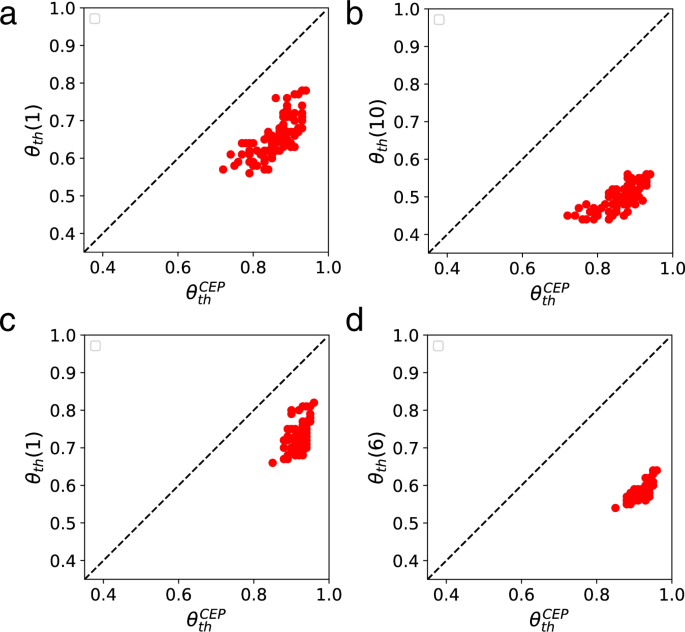Fig. 11: A comparison of the Fast ConPT algorithm and classical entanglement percolation (CEP).

## Conclusion

Table 1 summarizes the numerical results of our fast concurrence percolation theory (Fast ConPT) computation compared with previously known results24. The algorithm we have presented in this report utilizes two approximations to allow for numerical calculations of ConPT. Where available, our results are in good agreement with the analytical values of the concurrence percolation. We have also extended the analysis of the ConPT threshold to complex networks and demonstrated that our method could be applied to square lattices of 2002 nodes and complex networks of 300 nodes. Combining our method with more efficient path-counting algorithms would allow us to probe a more significant fraction of the total paths of a network for the ConPT calculation and provide a more robust estimate for the ConPT threshold. We believe that this work is an important step towards understanding the structural and communication properties of large-scale quantum networks.

We believe that ConPT is a promising tool for practically designing and analysing quantum networks. It offers crucial insights into how entanglement strength, viewed as a costly resource, should be distributed throughout a network to ensure resilient communication. In full-optical quantum communication networks, for example, entanglement strength is usually expressed as a function of the number of entangled photons shared between nodes39. Current methods used in simulations to determine the entanglement strength necessary for the emergence of a giant component in quantum networks or the connectivity of two random nodes implicitly assume the CEP measurement strategy, i.e., they assume that the topology of the quantum network is immutable like that of a classical network39,40. The numerical methods we have presented in this paper allow concurrence percolation theory to be practically useful in the analysis of large complex networks, providing a lower bound on the entanglement strength necessary for communication between distant nodes, therefore allowing the cost associated with establishing communication channels of a certain strength in these networks to be lowered. As we showed in our comparison with CEP, even for m = 1 the Fast ConPT method already predicts a lower entanglement percolation than CEP, demonstrating its effectiveness for determining how close any given measurement strategy is to being optimal.

Still, the critical behaviors of ConPT near θth remain an interesting and open question. Previous studies have indicated that some critical phenomena, such as the emergence of subgraphs, are drastically different in quantum random networks than in classical networks6. While Meng et al. has provided a finite-size analysis of the critical behaviors of Bethe lattices, their analysis of 2D lattices is limited by the size of the lattices they could investigate24. Their initial results indicate that the critical exponent ν associated with ConPT on 2D lattices are the same as those of classical percolation theory. This can now be investigated more thoroughly using the algorithm presented in the present work.